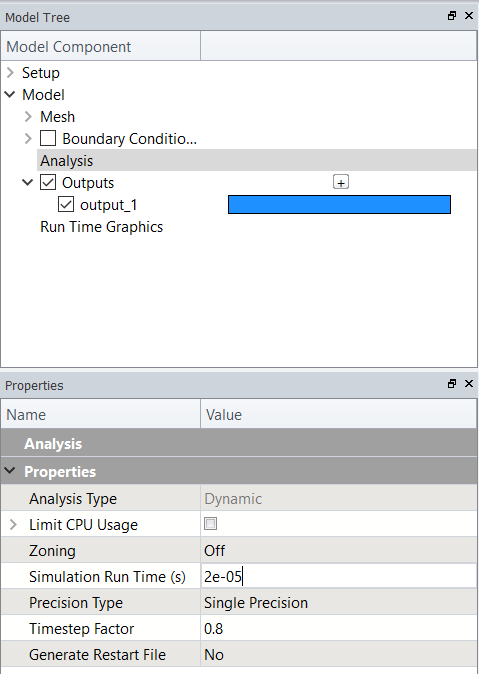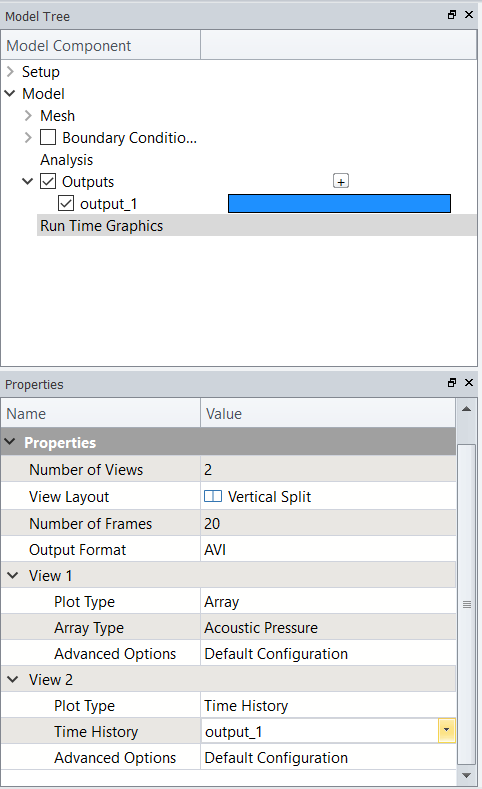# 5. Model Execution

Execution of a model can be as simple as one line of code. However, having the ability to control the specific outputs plotted during the simulation is useful for debugging purposes or verifying the model is running as expected.

## Execution Setup

Key information required prior to executing a model:

• Timestep
• Simulation Time - how long to execute model for
• Plot Settings - controlling plots during execution

## Timestep

The timestep is the maximum allowable transit time between 2 nodes to allow the OnScale solver to work efficiently. This is calculated in every model prior to execution. OnScale executes a model using an integer number of timesteps.

### Designer Mode

The process of calculating the timestep is automated within Designer Mode.

### Analyst Mode

The prcs command performs the timestep calculation based on your model setup (material properties, geometry and mesh) which can then be extracted using the symb #get operation:

```c process commandprcs
c execution setupsymb #get { step } timestep /* extract calculated timestep from prcs command```

## Simulation Time

The simulation time determines how long the model will run for, entered as a value in seconds.

### Designer Mode

Under the Analysis section in the model tree, the property window allows the value of simtime to be defined:### Analyst Mode

A variable simtime is defined to store the time value which we then use to calculate the equivalent simulation time in terms of number of timesteps:

`symb simtime = 40e-6 /* simulation time - 40 cycles @ 1 mhzsymb nexec = \$simtime / \$step /* determine how many timesteps to run full model`

## Plot Settings

We typically want to set up the data plots so that we can visualise what is happening during the execution. The plots are typically of any of the requested data fields or a requested time history.

### Designer Mode

The properties for Run Time allows a variety of plot settings to be controlled, including:

• Number of Views and View Layout
• Number of Frames - controls how often we update the data plotted during the execution period
• Individual View Settings:
• Plot Type - Time History or Data Field plot
• Time History - allows selection of specific time history data
• Array Type - allows selection of data field data### Analyst Mode

The optimal method of executing a model is by using a procedure. A procedure is a segment of code that can be recalled a number of times using the pcom command.

For controlling the plot settings, a grph command is issued to set up the views which we then plot to within the procedure.

```symb nloops = 40 /* plotting snapshots during model executionsymb nexec2 = \$nexec / \$nloops /* partition full simulation into smaller segments for plotting
c set up plottinggrph    arrow off /* turn off arrows    line off /* turn off mesh lines    set imag avi /* set image capture for movie file generation    nvew 3 1 /* set up 3 plotting windows in layout number 3    end
c create procedure - code that can be executed 'x' number of timesproc plot save /* name procedure 'plot' and save the code
exec \$nexec2 /* run model partially
grph    plot ydsp /* plot calculated data array    plot 1 /* plot time history 1 - drive function    plot 3 /* plot time history 3 - charge on top electrode    imag /* snapshot of graphics window for movie generation    endend\$ proc /* end of procedure code
c run model by calling 'plot' procedure 'nloops' timesproc plot \$nloopsterm```

Note that we have divided the total simulation timesteps to allow us to plot the calculated outputs during the execution until the full simulation time is reached.